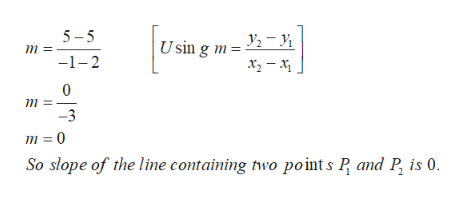# Find the slope of the line containing the given points. (If an answer is undefined, enter UNDEFINED.)P1(2, 2), P2(2, −3) Find the slope of the line containing the given points. (If an answer is undefined, enter UNDEFINED.)P1(2, 5), P2(−1, 5) Find the slope of the line containing the given points. (If an answer is undefined, enter UNDEFINED.)P1(−2, −4), P2(−3, −1)

Question
122 views

Find the slope of the line containing the given points. (If an answer is undefined, enter UNDEFINED.)

P1(2, 2), P2(2, −3)

Find the slope of the line containing the given points. (If an answer is undefined, enter UNDEFINED.)
P1(2, 5), P2(−1, 5)

Find the slope of the line containing the given points. (If an answer is undefined, enter UNDEFINED.)
P1(−2, −4), P2(−3, −1)

check_circle

Step 1

Slope m of the line containing two points A(x1 , y1) and B(x2 , y2) is

Step 2

a) Slope m of the line containing two points P1(2, 5) a...help_outlineImage Transcriptionclose5-5 Usin g m= 2-i m -1-2 0 -3 m 0 So slope of the line containing two point s P and P is 0. fullscreen

### Want to see the full answer?

See Solution

#### Want to see this answer and more?

Solutions are written by subject experts who are available 24/7. Questions are typically answered within 1 hour.*

See Solution
*Response times may vary by subject and question.
Tagged in

### Algebra